# 5.3. Applying Logic Rules¶

Computers generally work with bit patterns like 01011100 to represent information. That means that often we need to apply a rule like AND or OR to not just two-bits, but to two longer patterns of bits. To apply a rule like AND to two-bit patterns like 1010 and 1110, we simply apply the rule to each successive pair of bits in the two patterns as shown in the diagram below: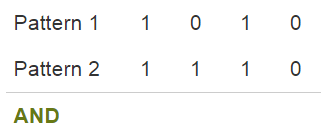We are going to apply the AND rule to combine the bit pattern 1010 with the pattern 1110. To do so, we will apply the rule to each “column” of bits in the two patterns.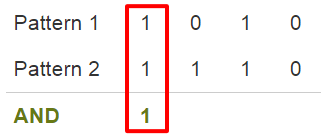The AND rule states that if both input bits are 1, the output is 1. The first bit of both patterns is 1, so we record a 1 for the first bit of our answer.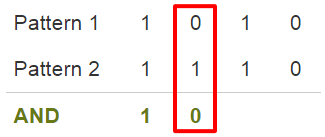Looking at the second bit of each pattern, we see a 0 in the first pattern and a 1 in the second. The AND rule says that the output is 0 unless both inputs are 1. So this means the second bit of our answer will be 0.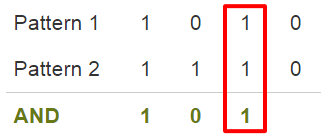A 1 in the third bit of both input patterns means the third bit of our answer is 1 as well.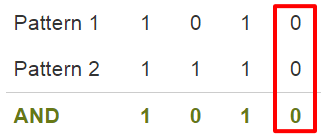Finally, in the last position, two 0’s result in 0 according to the AND rule. Our final answer is 1010. In any digit where both patterns had a 1 our answer has a 1; otherwise, it has a 0.

Here is the result of applying the OR rule to the same two patterns:

 Pattern 1 1 0 1 0 Pattern 2 1 1 1 0 OR 1 1 1 0
• First column: OR says that the result is 1 if either (or both) of the inputs is 1. So the first digit of the answer is a 1.

• Second column: Only the second pattern has a 1 in the second digit. But that is all the OR rule requires - one input to be 1. So the second answer digit is 1.

• Third column: Once again, two 1’s as input result in a 1 for our answer in the third digit.

• Fourth column: In the last digit, both patterns have 0’s. That is the only way that OR produces a 0. So our answer has a 0 in the fourth digit.